## Quadrilateral BECK is known to be a rhombus. Two of the vertices are B(3,5) and C(7,-3). a. Find one slope of diagonal EK b. Find

Question

Quadrilateral BECK is known to be a rhombus. Two of the vertices are B(3,5) and C(7,-3).
a. Find one slope of diagonal EK
b. Find an equation of line EK ​

in progress 0
3 months 2021-08-15T07:59:03+00:00 1 Answers 5 views 0

a. The slope of EK isb. The equation of line EK is y =x –Step-by-step explanation:

The form of the equation of a line is y = m x + b, where

• m is the slope of the line
• b is the y-intercept

The rule of the slope is m =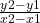, where

• (x1, y1) and (x2, y2) are two points on the line
• The rule of the mid-point is (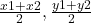)

BECK is a rhombus

∵ The diagonal is the line that joins two opposite vertices

∵ B and C are opposite vertices in the rhombus

∵ E and K are opposite vertices in the rhombus

BC and EK are the diagonals of the rhombus BECK

∵ The diagonals of the rhombus are ⊥ and bisect each other

EK is ⊥ bisector to BC

→ Let us find the slope and the mid-point of BC

∵ B = (3, 5) and C = (7, -3)

∴ x1 = 3 and y1 = 5

∴ x2 = 7 and y2 = -3

→ Substitute them in the rule of the slope above to find it

∵ m =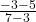=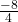= -2

∴ m = -2

The slope of BC = -2

→ To find the slope of EK reciprocal the slope of BC and change its sign

∴ m⊥ =∴ The slope of EK =a. The slope of EK is→ Substitute the value of the slope in the form of the equation above

∵ y =x + b

→ To find b substitute x and y in the equation by the coordinates

of a point on the line

∵ The mid-point of BC is the mid-point of EK

∵ The mid-point of BC = (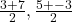) = (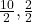) = (5, 1)

The mid-point of EK = (5, 1)

→ Substitute x by 5 and y by 2 in the equation

∵ 1 =(5) + b

∴ 1 =+ b

→ Subtractfrom both sides

∴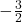= b

→ Substitute the value of b in the equation

∵ y =x +∴ y =x –b. The equation of line EK is y =x –